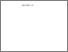# Mathematical and fuzzy modelling of high-speed interconnections in integrated circuits.

AHMAD, Tahir. (1998). Mathematical and fuzzy modelling of high-speed interconnections in integrated circuits. Doctoral, Sheffield Hallam University (United Kingdom)..Preview
PDF (Version of Record)
10694083.pdf - Accepted Version

## Abstract

Microstrip line are the most popular interconnection type mainly due to its planar geometry. The mode of propagation is almost a transverse electromagnetic mode of wave propagation (TEM) and can be described by the Telegrapher's equations. These facts make mathematical and fuzzy modelling of microstrip lines possible.Two types of nonuniformly coupled microstrip lines, namely, nonuniformly spaced and strictly nonuniform, are presented in this study. A new model of capacitance matrix was developed for nonuniformly spaced coupled microstrip lines. The model obtained was then translated into a Mathematica program in order to be utilised in real systems. Furthermore, a new matrix; mutual capacitance ratio matrix, was deduced from the previous model. A few valuable properties were then established from this matrix. Novel concepts were introduced to approximate capacitance of strictly nonuniform coupled microstrip lines and Mathematica programs were coded to implement these methods. The study then continued with the development of new algorithms to calculate the time delay and characteristic impedance using capacitance matrices of both types of nonuniform lines. These algorithms finally became a generalised algorithm which could be used in any type of coupled microstrip lines, uniform and nonuniform. The time delay and characteristic impedance were later used as parameters to simulate crosstalk using SPICE. Analysis of geometrical and electrical parameters of microstrip lines was performed mathematically and simulations modelled using the Mathematica package. Experimental work was also carried out to investigate the characteristic of crosstalk. All information obtained from these analyses were then fed into the developed novel fuzzy model. The model was designed to minimise crosstalk and to optimise the geometrical and electrical parameters of coupled microstrip lines simultaneously. These models have the potential to become 'multi purpose on board designing tools' for a designer before the system is finally fabricated.

Item Type: Thesis (Doctoral) Thesis (Ph.D.)--Sheffield Hallam University (United Kingdom), 1998. Sheffield Hallam Doctoral Theses EPrints Services 10 Apr 2018 17:18 26 Apr 2021 11:21 https://shura.shu.ac.uk/id/eprint/19203View Item